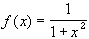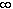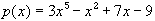#Interactive Real Analysis

## 6. Limits, Continuity, and Differentiation

### 6.4. Topology and Continuity

While the definition of continuity suffices for functions on the real line, there are other, more abstract spaces for which this definition will not work. In particular, our continuity definitions relies on the presence of an absolute value. There are spaces which do not have such a distance function, yet we still might want to study continuous functions on those abstract spaces. In this section we will investigate some topological properties of continuity which will, in fact, apply equally well to more general settings. In addition, this section will contain several important theoretical results on continuous function on the real line.
 Proposition 6.4.1: Continuity and Topology Let f be a function with domain D in R. Then the following statements are equivalent: f is continuous If D is open, then the inverse image of every open set under f is again open. If D is open, then the inverse image of every open interval under f is again open. If D is closed, then the inverse image of every closed set under f is again closed. If D is closed, then the inverse image of every closed interval under f is again closed. The inverse image of every open set under f is the intersection of D with an open set. The inverse image of every closed set under f is the intersection of D with a closed set.
This proposition can be used to prove that a function is continuous, and is especially nice if the domain of the function is either open or closed. This is true in particular for function defined on all of R (which is both open and closed).
 Examples 6.4.2:Let f(x) = x2. Show that f is continuous by proving that the inverse image of an open interval is open. that the inverse image of a closed interval is closed.Let f(x) = 1 if x > 0 and f(x) = -1 if x0. Show that f is not continuous by finding an open set whose inverse image is not open. finding a closed set whose inverse image is not closed.
Now we know that the inverse images of open sets are open, and the inverse images of closed sets are closed whenever f is continuous. What about the images of sets under continuous functions ?
 Examples 6.4.3:Is it true that if f is continuous, then the image of an open set is again open ? How about the image of a closed set ?
As the above examples show, the image of a closed set is not necessarily closed for continuous functions. It is also easy to see that the image of a bounded set is not necessarily bounded. However, the image of bounded and closed sets under continuous functions is both bounded and closed again. That is the content of the next theorem.
 Proposition 6.4.4: Images of Compact and Connected sets If f is a continuous function on a domain D, then: the image of every compact set is again compact. the image of every connected set is again connected.
Since compact sets in the real line are characterized by being closed and bounded, we should note while it is not true that the image of a closed set is closed, one must look at an unbounded closed set for a counterexample. If the set was closed and bounded, then its image would be closed again, because the image of a compact set is, in particular, closed, by the above theorem.
 Examples 6.4.5:Ifthen: what is the image of [-2, 1] ? find a closed set whose image is not closedFind examples for the following situations: A continuous function and a set whose image is not connected. A continuous function and a disconnected set whose image is connected. A function such that the image of a connected set is disconnected. Is it true that inverse images of connected sets under continuous functions are again connected ?
This proposition has several important consequences for continuous functions.
 Theorem 6.4.6: Max-Min theorem for Continuous Functions If f is a continuous function on a compact set K, then f has an absolute maximum and an absolute minimum on K. In particular, f must be bounded on the compact set K.
Note that the above example guaranties the existence of an absolute maximum and minimum, but does not indicate where that maximum or minimum might occur.
 Examples 6.4.7:Find a continuous function on a bounded interval that is unbounded. How about a continuous function on a bounded interval that does not have an absolute maximum but is bounded ?Does the functionhave an absolute maximum and minimum on [-2, 1] ? How about on the interval [0,)?
 Theorem 6.4.8: Bolzano Theorem If f is continuous on a closed interval [a, b] and f(a) and f(b) have opposite signs, then there exits a number c in the open interval (a, b) such that f(c) = 0.
 Examples 6.4.9:Show that the equation cos(x) = x has a solution in the interval [-10,10].Show that the equation= 0 has at least one solution in R.
 Theorem 6.4.10: Intermediate Value Theorem If f is continuous on a closed interval [a, b] and d is any number between f(a) and f(b). Then there exists a number c in the open interval (a, b) such that f(c) = d.